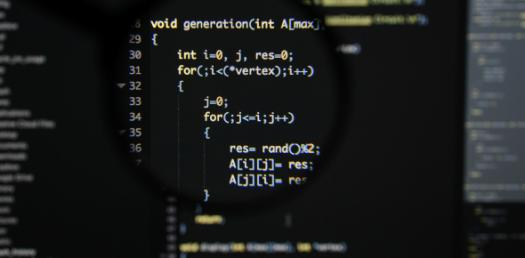# C Programming Basics - Functions, Arrays, Loops, ETC.

Approved & Edited by ProProfs Editorial Team
At ProProfs Quizzes, our dedicated in-house team of experts takes pride in their work. With a sharp eye for detail, they meticulously review each quiz. This ensures that every quiz, taken by over 100 million users, meets our standards of accuracy, clarity, and engagement.
| Written by ProgrammerJAJ
P
ProgrammerJAJ
Community Contributor
Quizzes Created: 1 | Total Attempts: 347
Questions: 10 | Attempts: 347SettingsC programming language is a robust language with a rich set of built in functions and operators that can be used to write any complex program. Take up the C programming basics- functions, array, loops etc. Quiz below and get to understand some basic C programing skills. All the best!

• 1.

• A.

True

• B.

False

A. True
Explanation
To include a header file in a C++ program, the correct syntax is #include "headerfile.h". This line of code tells the compiler to insert the contents of the specified header file into the program at that point. Therefore, the statement "To declare a header file you use #include headerfile.h" is true.

Rate this question:

• 2.

### The printf function is located in the following header files:

• A.

Stdio.h

• B.

Stdlib.h

• C.

Math.h

• D.

Iostream

• E.

Cmath

• F.

Cstdlib

A. Stdio.h
D. Iostream
Explanation
The correct answer is stdio.h and iostream. These two header files contain the necessary functions and declarations for input and output operations in C and C++. stdio.h is the standard input/output library in C, while iostream is the input/output stream library in C++.

Rate this question:

• 3.

### To declare a constant variable you use the following statement:

• A.

Constant

• B.

Cont

• C.

Const

• D.

Conts

• E.

Cos

C. Const
Explanation
The correct answer is "const". In programming, the "const" keyword is used to declare a constant variable, which is a variable whose value cannot be changed once it is assigned. By using "const", you can ensure that the value of the variable remains constant throughout the program, preventing accidental modifications and providing clarity to other programmers who read the code.

Rate this question:

• 4.

### Which is the correct way to declare an array?

• A.

Int a;

• B.

A char;

• C.

A int;

• D.

A[int];

A. Int a;
Explanation
The correct way to declare an array is "int a;". This statement declares an array named "a" of size 12, where each element is of type integer. The square brackets "[]" are used to indicate that "a" is an array, and the number inside the brackets specifies the size of the array.

Rate this question:

• 5.

### The following is a correct do while statement: do{ printf("enter a number: "); scanf("%d", &i); } while(i <= 12)

• A.

True

• B.

False

B. False
Explanation
The programmer forgot the semicolon ( ; )

should be like so:

do{
statements;
}while(expression);

Rate this question:

• 6.

### Is this correct? #include int main() { int i = 0; int j = 2; if(i < j) printf("i variable is smaller than j variable"); return 0; }

• A.

True

• B.

False

A. True
Explanation
The given code snippet is correct. It includes the necessary header file, defines the main function, and declares and initializes two integer variables i and j. The if statement checks if i is less than j, and if it is, it prints the message "i variable is smaller than j variable". Since the value of i is 0 and the value of j is 2, the condition i < j is true, and the message is printed. Finally, the main function returns 0 to indicate successful execution. Therefore, the correct answer is True.

Rate this question:

• 7.

### The pow function is located in the _______ header file

math.h
cmath
Explanation
The pow function is located in the math.h and cmath header files. These header files are used in C and C++ programming languages to include mathematical functions and constants. The pow function specifically is used to calculate the power of a number, where the first argument is the base and the second argument is the exponent. By including the math.h or cmath header file, developers can access and use the pow function in their code.

Rate this question:

• 8.

### A return statement returns a value to the function from which it is called from

• A.

True

• B.

False

A. True
Explanation
A return statement in programming is used to end the execution of a function and return a value back to the function that called it. This allows the function to pass data or results back to the calling function. Therefore, the given statement that a return statement returns a value to the function from which it is called from is true.

Rate this question:

• 9.

### C is an Object Oriented Programming Language

• A.

True

• B.

False

B. False
Explanation
The given statement "C is an Object Oriented Programming Language" is false. C is not an object-oriented programming language. It is a procedural programming language that focuses on procedures and functions rather than objects and classes. Object-oriented programming languages like C++ and Java have features like encapsulation, inheritance, and polymorphism, which C lacks.

Rate this question:

• 10.

### Learning C is a good way to learn C++

• A.

True

• B.

FalseBack to top